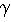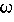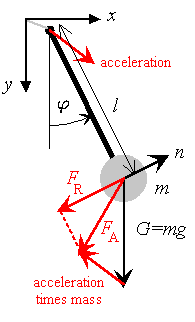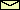# Equations of Motionof damped and driven pendula

The derivation of the equations of motion of damped and driven pendula extends the derivation of the undamped and undriven case. Damping and driving are caused by two additional forces acting on the pendulum: The damping force and the driving force.

## Damping force

An undamped pendulum can be realized only virtually as here in the Pendulum Lab. In reality dissipation of energy leading to damping is unavoidable. Usually dissipation is included in the equation of motion by adding a viscous damping term which is a damping constant times the velocity. Thus, the equation of motion of the damped pendulum reads

 (1) d2/dt2 +d/dt +02sin= 0,

whereis the damping constant. Such a force occurs, for example, when a sphere is dragged through a viscous medium (a fluid or a gas). For a laminar flow (i.e., a flow without eddies) the dragging force is given by Stoke's law Fdamping = -6Rv, whereis the viscosity of the medium, R is the radius of the sphere, and v is its velocity relative to the medium.

In general, different damping mechanisms of different strengths are possible. Imagine, e.g., a heavy spherical mass which hangs at a thin wire rope from the ceiling. Here, the main sources of damping are aerodynamical friction due to the motion of the mass through the air and friction caused by bending the rope at the suspension point. Aerodynamical friction follows Stoke's law only for very small velocities. For large velocities, when the flow becomes turbulent, the friction force increases roughly quadratically. The friction of the rope is caused by plastic deformation and solid friction if it is made by many fibres. In both cases, the friction force may dependent on the velocity and other parameters in a complicated way. In general, a viscous damping force is usually only a phenomenological force. Even if it is empirically correct, it is often difficult to calculate the damping constant. Here in the Pendulum Lab, the damping force is always the viscous damping termd/dt.

## Driving force

There are several ways to drive a pendulum. The most simplest one is to add a periodic force. This leads to

 (periodic force) d2/dt2 +d/dt +02sin= (A/l)02cos 2ft,

where A and f are the amplitude and the frequency of driving. The amplitude is a length. In the lab the driving is visualized by a rubber string of zero equilibrium length. It has a fictitious spring constant of m02. The rubber is stretched periodically. The maximum elongation is just A.Instead of applying a periodic force, one can also drive the pendulum by moving its suspension point. Of course, only accelerated or curved motions lead to a driving force. In the framework moving with the suspension point, a mass feels any acceleration as a pseudo force FA which is the mass times the negative acceleration of the suspension point in the laboratory frame. Thus, the total force due to the acceleration of gravity and the acceleration of the suspension point reads

FA = -md2x0/dt2 ex + m(g-d2y0/dt) ey,

where x0(t) and y0(t) are the coordinates of the suspension point and ex and ey are the unity vectors in x and y direction, respectively. The restoring force FR is the projection of FA onto the unity vector n = cosex - siney which is tangential at the circle of radius l. Thus,

FR = n FA = -md2x0/dt2 cos- m(g-d2y0/dt)sin.

Therefore, the equation of motion of a damped pendulum driven by an arbitray motion of its suspension point reads

 (2) d2/dt2 +d/dt +02sin= -d2(x0/l)/dt2 cos+ d2(y0/l)/dt2 sin.

Three different kind of periodic motions of the suspension point are realized in the Pendulum Lab:

horizontal motion:
x0 = A cos 2ft,
y0 = 0.
vertical motion:
x0 = 0,
y0 = A cos 2ft.
rotation:
x0 = A sin 2ft,
y0 = A cos 2ft.

The corresponding equations of motion read:

 (horizontal motion) d2/dt2 +d/dt +02sin= a cos(2ft) cos,

 (vertical motion) d2/dt2 +d/dt +02sin= -a cos(2ft) sin,

and

 (rotation) d2/dt2 +d/dt +02sin= a sin(2ft-),

respectively, where
 (3) a = (2f)2 A/l.

 QUESTIONS worth to think about: Think about a pendulum built by a nearly massless string and spherical mass swinging in air. Neglect damping due to bending of the string. Does the damping constantdepend on the mass m of the sphere? How large is it? How large is the energy of the pendulum in terms of angle, angular velocity, and phase of external driving.
© 1998 Franz-Josef Elmer,Franz-Josef doht Elmer aht unibas doht ch, last modified Sunday, July 19, 1998.